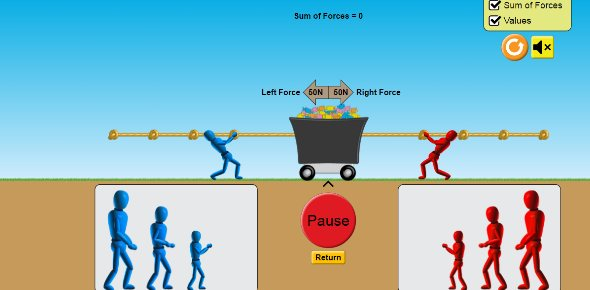# Interaction Of Force And Motion Quiz

10 Questions | Total Attempts: 157SettingsCovers the 7 parts of Interaction of Force and Motion. Contact Force Distance Force Net Force Graphing Speed Calculating Speed Acceleration Newton's Laws

• 1.
What is acceleration? Answer in the box below, in complete sentences.
• 2.
Write a response to the Prezi you saw today, what you learned, what you already knew, and a little bit about the conclusion. Be sure to write in complete sentences!
• 3.
Which of the following is an example of a Distance Force?
• A.

Holding a book in your hand.

• B.

A boy riding a bike.

• C.

Gravity from the earth pulling you down.

• D.

A car pulling a trailer.

• 4.
A Net Force is a force between two objects that are touching one another.
• A.

True

• B.

False

• 5.
Which is the first law out of the three of Newton's laws?
• A.

Any object at rest will stay at rest, unless it's acted upon by an unbalanced force. Any object in motion will continue to stay in motion unless it's acted upon by and unbalanced force. This law is often called the law of inertia.

• B.

Every action has and equal opposite re-action.

• C.

Acceleration may be produced when force acts on a mass. The bigger the mass, the greater the force needed to act on it.

• 6.
Where does the time (independent variable) go when graphing speed?
• A.

Y axis

• B.

The line graphing the objects data.

• C.

None

• D.

X axis

• 7.
To calculate speed, you take the _______ and _____ it by the ______. Type in the letter next to the word, example: c. cat, b. elephant, a. giraffe  The __c.___ meowed at the dog.
• 8.
My rate would be 2.89 miles an hour if my distance was 3.33 miles and my time was 1hr 15 min.
• A.

True

• B.

False

• 9.
What are the interactions?
• A.

Contact Force, Acceleration, Net Force, Graphing Speed, Distance Forces, Newton's Laws, Friction.

• B.

Contact Force, Acceleration, Net Force, Graphing Speed, Calculating Speed, Distance Forces, Newton's Laws.

• C.

Contact Force, Acceleration, Net Force, Graphing Speed, Calculating Speed, Newton's Laws, Conservation.

• 10.
A ______ Force is where you take the _____ of all the forces acting upon one object.
Related TopicsBack to top